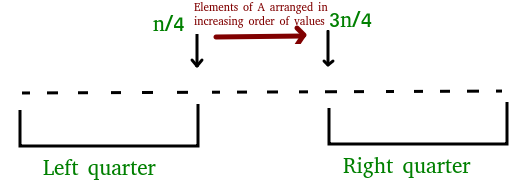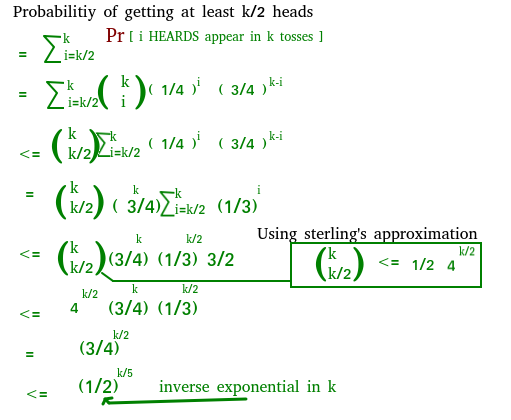# Randomized Algorithms | Set 3 (1/2 Approximate Median)

• Difficulty Level : Basic
• Last Updated : 19 Sep, 2018

We strongly recommend to refer below articles as a prerequisite of this.

In this post, a Monte Carlo algorithm is discussed.

Problem Statement : Given an unsorted array A[] of n numbers and ε > 0, compute an element whose rank (position in sorted A[]) is in the range [(1 – ε)n/2, (1 + ε)n/2].
For ½ Approximate Median Algorithm &epsilom; is 1/2 => rank should be in the range [n/4, 3n/4]

We can find k’th smallest element in O(n) expected time and O(n) worst case time.

What if we want in less than O(n) time with low probable error allowed?
Following steps represent an algorithm that is O((Log n) x (Log Log n)) time and produces incorrect result with probability less than or equal to 2/n2.

1. Randomly choose k elements from the array where k=c log n (c is some constant)
2. Insert then into a set.
3. Sort elements of the set.
4. Return median of the set i.e. (k/2)th element from the set
5. ## C

 `/* C++ program to find Approximate Median using ` `   ``1/2 Approximate Algorithm */` `#include ` `using` `namespace` `std; ` ` `  `// This function returns the Approximate Median ` `int` `randApproxMedian(``int` `arr[],``int` `n) ` `{ ` `    ``// Declaration for the random number generator ` `    ``random_device rand_dev; ` `    ``mt19937 generator(rand_dev()); ` ` `  `    ``// Random number generated will be in the range [0,n-1] ` `    ``uniform_int_distribution<``int``> distribution(0, n-1); ` ` `  `    ``if` `(n==0) ` `        ``return` `0; ` ` `  `    ``int` `k = 10*log2(n); ``// Taking c as 10 ` ` `  `    ``// A set stores unique elements in sorted order ` `    ``set<``int``> s; ` `    ``for` `(``int` `i=0; i ::iterator itr = s.begin(); ` ` `  `    ``// Report the median of the set at k/2 position ` `    ``// Move the itr to k/2th position ` `    ``advance(itr, (s.size()/2) - 1); ` ` `  `    ``// Return the median ` `    ``return` `*itr; ` `} ` ` `  `// Driver method to test above method ` `int` `main() ` `{ ` `    ``int` `arr[] = {1, 3, 2, 4, 5, 6, 8, 7}; ` `    ``int` `n = ``sizeof``(arr)/``sizeof``(``int``); ` `    ``printf``(``"Approximate Median is %d\n"``,randApproxMedian(arr,n)); ` `    ``return` `0 ` `} `

## Java

 `/* Java program to find Approximate Median using ` `   ``1/2 Approximate Algorithm */` `import` `java.util.Iterator; ` `import` `java.util.Random; ` `import` `java.util.TreeSet; ` ` `  ` `  `class` `Test ` `{ ` `    ``static` `int` `arr[] = ``new` `int``[]{``1``, ``3``, ``2``, ``4``, ``5``, ``6``, ``8``, ``7``} ; ` `     `  `    ``// This function returns the Approximate Median ` `    ``static` `int` `randApproxMedian(``int` `n) ` `    ``{ ` `        ``// Declaration for the random number  ` `        ``Random r = ``new` `Random(); ` `         `  `        ``if` `(n==``0``) ` `            ``return` `0``; ` `         `  `        ``double` `k1 = ``10``*Math.log(n); ``// Taking c as 10 ` `      `  `        ``int` `k = (``int``)k1; ` `         `  `        ``// A treeset stores unique elements in sorted order ` `        ``TreeSet s = ``new` `TreeSet(); ` `        ``for` `(``int` `i=``0``; i itr = s.iterator(); ` `         `  `        ``int` `temp = s.size()/``2` `- ``1``; ` `         `  `        ``for` `(``int` `i = ``0``; i < temp; i++) { ` `            ``itr.next(); ` `        ``} ` `      `  `        ``// Return the median ` `        ``return` `itr.next(); ` `    ``} ` `  `  `    ``// Driver method to test the above function ` `    ``public` `static` `void` `main(String[] args) { ` `     `  `        ``System.out.println(``"Approximate Median is "` `+ randApproxMedian(arr.length)); ` `     `  `    ``} ` `} `

Output:

`Approximate Median is 4`

Time Complexity:
We use a set provided by the STL in C++. In STL Set, insertion for each element takes O(log k). So for k insertions, time taken is O (k log k).
Now replacing k with c log n
=>O(c log n (log (clog n))) =>O (log n (log log n))

How is probability of error less than 2/n2?
Algorithm makes an error if the set S has at least k/2 elements are from the Left Quarter or Right Quarter.It is quite easy to visualize this statement since the median which we report will be (k/2)th element and if we take k/2 elements from the left quarter(or right quarter) the median will be from the left quarter (or the right quarter).

An array can be divided into 4 quarters each of size n/4. So P(selecting left quarter) is 1/4. So what is the probability that at least k/2 elements are from the Left Quarter or Right Quarter? This probability problem is same as below :

Given a coin which gives HEADS with probability 1/4 and TAILS with 3/4. The coin is tossed k times. What is the probability that we get at least k/2 HEADS is less than or equal to?

Explanation:```If we put k = c log n for c = 10, we get
P <= (1/2)2log n
P <= (1/2)log n2
P <= n-2
```

Probability of selecting at least k/2 elements from the left quarter) <= 1/n2
Probability of selecting at least k/2 elements from the left or right quarter) <= 2/n2

Therefore algorithm produces incorrect result with probability less that or equal to 2/n2.

This article is contributed by Chirag Agarwal. If you like GeeksforGeeks and would like to contribute, you can also write an article and mail your article to contribute@geeksforgeeks.org. See your article appearing on the GeeksforGeeks main page and help other Geeks.